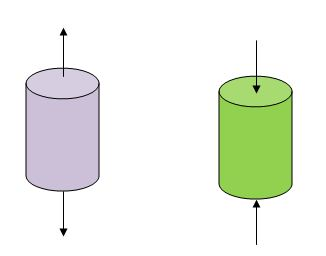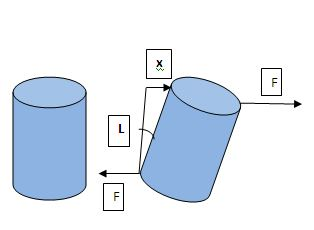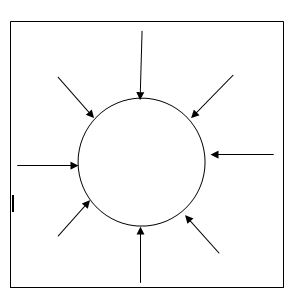# PhysicsTeacher.in

## High School Physics BLOG - online class notesRSS Feed

# Hooke’s Law – Modulus of Elasticity – stress and strain

In our last post we have discussed on and Plasticity. Here we will continue with that discussion and gradually cover the Hooke’s Law and Modulus of Elasticity. On the way we will learn two more terms: stress and strain. Finally we will cover (a) Young’s Modulus (2) Shear Modulus (3) Bulk modulus. So let’s start.

## Stress

To understand the Hooke’s Law it’s mandatory to understand 2 terms: Stress and Strain. Lets get some idea about those.
Let a force is applied on a body which can modify the shape and size of the object. Let’s call that Deforming Force as F.

As per Newton’s 3rd Law, a restoring force (say F1) will be generated inside which is equal but opposite of the deforming force.
F1 = -F.  Here F is the action and F1 is the reaction force.

The restoring force per unit area is called stress. Considering only the magnitude of the restoring force, we can write the expression of stress as:
Stress = F / A, where A = area of cross section of the body where action – reaction forces are being applied. Its unit in SI is Newton/meter^2   or PASCAL.

## Stress and Strain

A solid may change its dimensions is 3 different ways, resulting in 3 types of Stress and strain.

###### Tensile and Compressive stress (also cumulatively known as Longitudinal stress)

Here forces are applied at right angle to the cross sectional area or surfaces to either elongate or compress the object. That means these forces tend to cause change in length of the solid body and generates Longitudinal Stress. As said this stress may be of 2 types, tensile and compressive.longitudinal stress – elongate / compress

Tensile stress tends to enhance length and compressive stress tries to decrease the length.

Longitudinal Stress = Force applied / area of the surface = F/A

Longitudinal Strain:

Say the original length is L and change in length is l. Here comes the term Longitudinal Strain which is stated as the change in length per unit length.

So Longitudinal Strain = l/L……………………..(1)

### Tangential or Shearing Stress

If 2 equal and opposite deforming forces are applied parallel to the cross sectional area of a cylinder like object, then there is a relative displacement between the opposite faces of the cylinder.

In this case, the restoring force per unit area due to the applied tangential force is called tangential or shearing stress.

Shearing stress = F/A.

#### Shearing Strain

Related strain is called shearing strain.

In the diagram, 2 equal, opposite and parallel forces with magnitude F cause a relative displacement x between the opposite faces of the cylinder.
And during this displacement an angle θ is generated between the original and displaced side lines.

Here Shearing Strain = x/L = tan θ.   ………………… (2)
If this angle θ is too small, then tan θ = θ ……………. (3)
so from (2 & 3),  Shearing Strain = x/L = θ ……………….(4)shearing stress

### Hydraulic Stress

When a solid (say sphere) is placed in fluid under high pressure and compressed uniformly on all sides, then the force applied by the fluid acts in perpendicular direction at each point of the surface.
This is called Hydraulic Compression. This leads to decrease in volume.hydraulic stress

The internal restoring force per unit area is called Hydraulic Stress.
Hydraulic stress = F/A = hydraulic pressure.

#### Volume Strain

Related strain is called volume strain which is the ratio of change in volume and initial volume.
Volume Strain = Δv / V …………………. (5)

Next, we will state and discus the Hooke’s Law.

## Hooke’s Law

Hooke’s Law of Elasticity states that: For small deformations, stress is proportional to strain.

Stress ∝ strain

So, Stress = K. Strain     [ Hooke’s Law Equation ]  …………………….  (a)
here K is a constant.

## Modulus of Elasticity

From equation (a) we get, Stress/Strain = K ……………………. (b)

This K is a proportionality constant called Modulus of Elasticity.

From equation 2, we can say that Modulus of Elasticity is the ratio of Stress and Strain.

Now considering 3 different types of stress for solid, we have 3 different sets of elasticity modulus.

They are (a) Young’s Modulus (2) Shear Modulus (3) Bulk modulus

## 3 different sets of elasticity modulus

### Young’s Modulus

Young’s Modulus is the ratio of Longitudinal Stress and Longitudinal Strain. If it’s designated with Y then

Y = Longitudinal Stress / Longitudinal Strain = (F/A)/(l/L) = (FL)/(Al)

Its unit is N/m^2 or Pascal. Please note that Strain is dimensionless.

### Shear Modulus

Shear Modulus is the ratio of Shear Stress and Shear Strain. This is also known as Modulus of Rigidity.

If it’s designated as G then

G = (F/A)/(x/L) = (F/A)/θ = F/(Aθ).

### Bulk Modulus

Bulk Modulus (B)is the ratio of Hydraulic Stress and Volume Strain.

B = (F/A)/(Δv / V) = – P /(Δv / V)

Here P is the pressure. As the change in volume is indirectly proportional to the pressure applied, that’s why the negative sign is used.